# [翻译原论文/paper translation] ULMFiT for Text Classification

Recently I was struggled with a text classification related work, and accidentally came across fastai which is a very useful library, so I started to study the courses. Although I have finished Part1’s videos and lesson10 of 2018 courses, I still only have a vague idea about the fastai underlying work, so I plan to intensively read the original ULMFiT paper published in 2018 ACL and try to do a Chinese translation.
Welcome everyone to correct and discuss!

1 Like

# Universal Language Model Fine-tuning for Text Classification

Contributions

## 2. Related work

Transfer learning in CV

Hypercolumns

Fine-tuning

## 3. Universal Language Model Fine-tuning

ULMFiT包括以下步骤，如图1所示：1）通用域 LM 预训练；2）目标任务 LM 微调；3）目标任务分类器微调。我们将在之后的章节讨论这些内容。

## 3.2 Target task LM fine-tuning

Discriminative fine-tuning

\theta_{t}=\theta_{t-1}-\eta \cdot \nabla_{\theta} J(\theta)

\theta_{t}^{l}=\theta_{t-1}^{l}-\eta^{l} \cdot \nabla_{\theta^{l}} J(\theta)

Slanted triangular learning rates

cut=\left\lfloor T \cdot cut_{-}frac\right\rfloor
p=\left\{\begin{array}{ll}{t/cut,} & {\text {if } t<cut} \\ {1-\frac{t-cut}{cut \cdot(1/cut-frac-1)},} & {\text{otherwise}}\end{array}\right.
\eta_{t}=\eta_{\max} \cdot \frac{1+p \cdot(\text {ratio}-1)}{\text {ratio}}

STLR 以增长期短、衰减期长的方式更改三角学习率，我们发现这是取得良好性能表现的关键。在第 5 章中，我们将与过激的余弦退火相比较，那是一个类似的方法，并且已在 CV 中取得了最佳性能表现。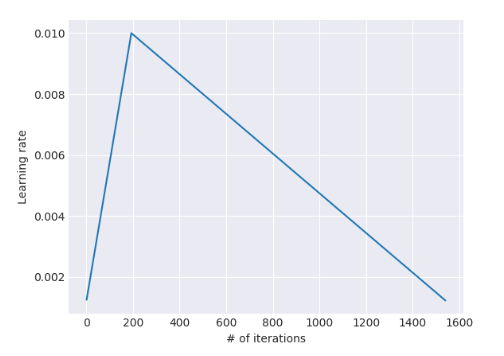## 3.3 Target task classifier fine-tuning

Concat pooling

\mathbf{h}_{c}=\left[\mathbf{h}_{T}, \operatorname{maxpool}(\mathbf{H}), \operatorname{meanpool}(\mathbf{H})\right]

BPTT for Text Classification (BPT3C)

Bidirectional language model

## 4.1 Experimental setup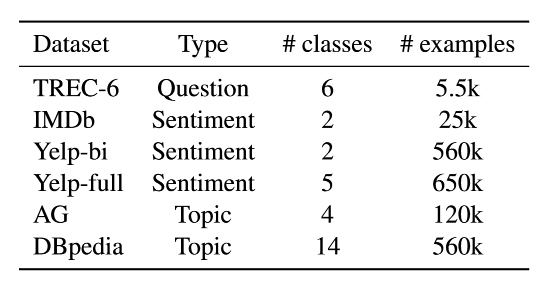Sentiment Analysis

Question Classification

Topic classification

Pre-processing

Hyperparameters

Baselines and comparison models

## 5. Analysis

Low-shot learning

Impact of pretraining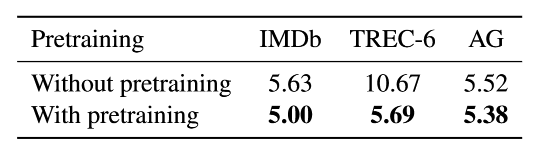Impact of LM quality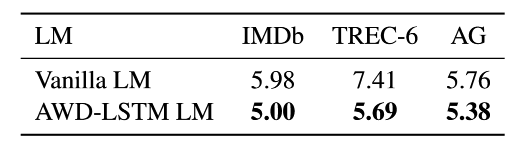Impact of LM fine-tuning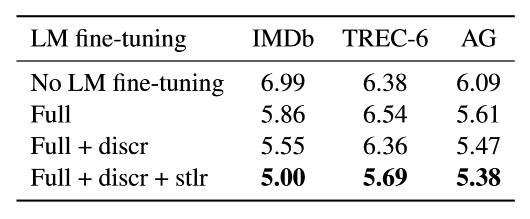Impact of classifier fine-tuning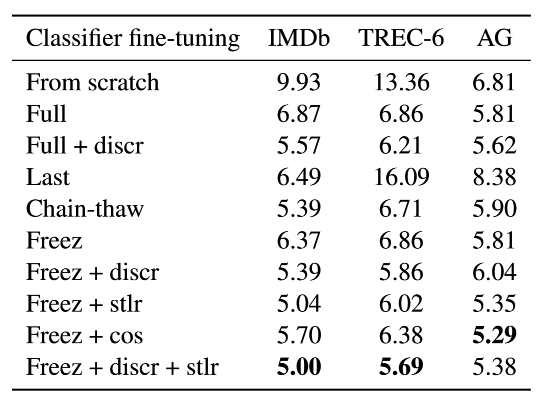Classifier fine-tuning behavior

Impact of bidirectionality

## 7. Conclusion

Translation completed. Sincerely hope corrections and suggestions!

1 Like

Very cool @thousfeet! Hey @sebastianruder have a lookThanks!https://mathpix.com/ 用这个可以直接截图导出LaTex代码，超级好用，不管是自己画得，视频里的还是手机拍个图，比贴图好看多了

1 Like